Next: Breaking Knapsack Cryptosystems Up: Subset-Sum (Knapsack) problems and Previous: Subset-sum problems are hard   Contents

## A proposed public-key system based on subset-sum

As an example of an easy subset sum problem, consider the task of determining what subset of(1)

adds up to 836. Thein this problem have the special property that every number is greater than the sum of all preceding numbers (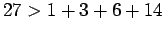, etc). [A sequence with this property is called super-increasing.]

1400 clearly cannot be in the set. If 650 is not in the set, we would be in trouble, since the sum of the remaining numbers is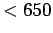, hence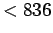. Thus 650 must be in the set, and we now have the task of finding numbers which add up to. 300 is too big, and the same reasoning as before shows that 150 must be in the set. If we continue, it is easy to identify the desired set as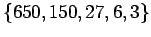.

We began section 4.1 with the problem of identifying a subset of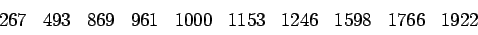which adds up to 5842. What we didn't mention before was that thewere carefully chosen to make them directly related to thein the easy subset-sum problem (1):and so forth, where 2039 is a modulus chosen in advance (larger than any of the numbers in the easy subset-sum problem) and 1000 is an arbitrarily chosen number. (we must have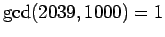)

To find the subset, begin by solving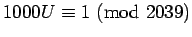, which gives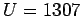. If we letbe the numbers in the easy problem, the hard problem can be written as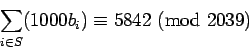When we multiply by 1307, this becomesIt is easy to identify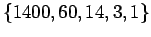as a subset which adds to 1478, and the desired subset of the original system isThis would seem to give us a good public-key system: a problem which is easy once some special information (the 2039 and the 1000) is known, difficult without the information. Unfortunately, the special type of subset-sum problem created in this way can be solved even without the special information. There is a sequence of papers showing how to solve special subset-sum problems and proposing a refinement which, in turn, was solved by the next paper in the sequence. This has not happened with the RSA system, but there is no guarantee that it won't!Next: Breaking Knapsack Cryptosystems Up: Subset-Sum (Knapsack) problems and Previous: Subset-sum problems are hard   Contents
Translated from LaTeX by Scott Sutherland
2002-12-14